# Algebra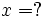This article/section deals with mathematical concepts appropriate for a student in early high school.

Algebra is a major branch of mathematics that analyzes the relationships between quantities or items. In higher math the principal fields of algebra are linear algebra, which focuses on matrices, and abstract algebra, which includes group theory.

## Elementary Algebra

While algebra is a very wide-ranging and advanced topic in theoretical mathematics, the word also refers to a very important topic in middle-school and high-school mathematics. This topic, which is sometimes known as "elementary algebra" to distinguish it from the more advanced branches, is the handling of arithmetical expressions and operations involving quantities that aren't known numbers, but are symbols like x, y, or z. These symbols are generally referred to as "variables" or "unknowns".

Whereas, in elementary arithmetic a typical question might be:

What is the value of 3+4?

in elementary algebra a typical question might be:

If x+4 = 7, what is the value of x?

Of course this example is so simple as to be practically pointless—why would someone ask a question this way?—the use of algebra opens up sophisticated ways of solving sophisticated problems.

Before continuing, let's see how we actually solved that. We subtracted 4 from each side of the equation. On the left side, subtracting 4 canceled the "+4", so we got "x+4-4", which is, of course, just x. On the right side, we got 7-4, which is 3. We used the principle that

You can apply mathematical operations to something, even if it contains unknowns like "x".

The goal was to get an equation that has just "x" on the left side, with some number on the right. In the original problem, x was on the left side, but hidden with some other mathematical operations. Our job was to strip off those operations, one at a time. Here's a more complicated example: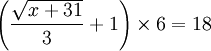We undo the multiplication, by dividing both sides by 6: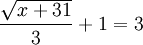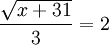We undo the division:We undo the square root: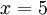As one gets more skillful at these kinds of manipulations, one can handle more complex problems. A typical case involves an equation in which the unknown appears in more than one place. After stripping away as many operations as we can, we might be left with something like: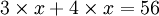At this point we use the distributive law, along with the principle that

The various laws of mathematics (commutative, associative, distributive) work even when the items appearing in the expressions are unknowns.

The distributive law tells us thatso we get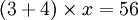and hence x = 8. You can plug x = 8 intoand see that the result is 24+32, or 56.

## Polynomial Manipulations

As one's proficiency increases, one can handle increasingly complicated equations. The next step involves things like this: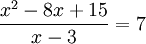This can be solved if one knows that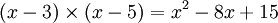sofrom which one gets x = 12.

This was just a case of the principle that you can perform arithmetic operations even when the expression contain unknowns. In this case we were actually applying the distributive law 3 times.When we multiply the sum of two things times the sum of two things, we get the sum of four things. This is an extremely common operation, and there is a mnemonic for it—"FOIL"—which stands for "first, outside, inside, last". We multiply the first item in each parenthesized expression; that's x times x. We multiply the "outside" items; that's x times -5. The "inside" items are -3 times x, and the "last" items are -3 times -5.

This same principle applies when there are more than two items in each factor; it just doesn't have a useful mnemonic word. Each term in the first factor (first parenthesized expression) get multiplied by each term in the second factor, and all the products are added up.

A quadratic equation is a type of equation in which the degree of x is exactly 2. Usually, these equations have two possible values of x. An example of a quadratic equation would be:There are several ways to solve quadratic equations. Let's look at three of the most common and use each to solve the above equation.

### Factoring

Factoring is a method of solving quadratic equations that involves factoring the quadratic expression into smaller parts, and solving each part individually. It's often the fastest way to solve quadratics, if you can "see" the factors easily. In the case of our above equation, we can indeed factor it: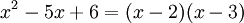Substituting the factored expression into the original equation yields:The key here is to recognize that if multiplying two or more numbers gives a result of zero, at least one of those numbers must itself equal zero. (It is impossible for there to be two nonzero numbers whose product is zero.) So the above equation can be split into two equations:Thus x must equal either 2 or 3. There is no way to tell which is the "real" value of x, so the actual answer is written as: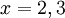This is just shorthand for "x is either 2 or 3."

### Completing the square

Completing the square is a method of solving quadratic equations that involves turning the left-hand side of the equation into a perfect square trinomial and then taking the square root of both sides. In our original equation:the left-hand side is not a perfect square trinomial. That is to say, if we tried to take the square root of it, we couldn't simplify it any further, so that wouldn't help us in solving the equation. We need to change the equation to make it a perfect square.

The key here is the coefficient of the linear term, which is the number that's multiplied by the x that isn't squared. For us, that's 5. We divide that number by 2 and then square it, which yields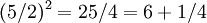So if we add 1/4 to both sides, the left side will be a perfect square trinomial. Thus our new equation is: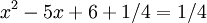Or, equivalently: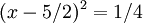We can now take the square root: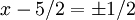The "±" indicates that the 1/2 is either positive or negative. The result is thus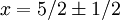Since 5/2 + 1/2 = 3, and 5/2 - 1/2 = 2, this is equivalent to the result obtained through factoring.

## Notes and references

1. The name algebra comes from the Arabic word al jebr, which means reduction or "reunion of broken parts" Algebra as mentioned in a book Hisab al-jabr w'al-muqabala translated as Science of the Reunion and the Opposition. This text was written in in about 830 AD by Mohammad ibn-Musa al-Khwarizmi of Baghdad. See the Biography of Al-Khwarizmi